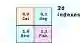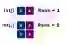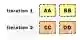# C# 2D Array Examples

Allocate, loop over and add to 2D arrays. Iterate over elements with nested for-loops.

2D array. A glowing platform appears at the water's edge. The platform is solid. You step onto it. Suddenly an entire dimension is illuminated—a new world is revealed.Array notes. In this 2D world, an element is addressed with X and Y. Consider now C#: 2D arrays can store any element type. Jagged arrays can also represent our 2D space.Jagged Arrays

First example. Here we show a 2-dimensional string array. The program creates a 2x2 string array and then prints out all 4 elements with Console.WriteLine.
Part A: Here we initialize the 2D array with an initializer expression. Each element in this array is a string.
Part B: We use the indexing syntax to access elements. We use 2 indexes for a 2D array. Each dimension is indexed starting at zero.
Rows, columns: It makes no difference whether we think of the first index as a row or column, or the other way around.C# program that creates 2D array using System; class Program { static void Main() { // Part A: create 2D array of strings. string[,] array = new string[,] { {"cat", "dog"}, {"bird", "fish"}, }; // Part B: access (and print) values. Console.WriteLine(array[0, 0]); Console.WriteLine(array[0, 1]); Console.WriteLine(array[1, 0]); Console.WriteLine(array[1, 1]); } } Output cat dog bird fish

Rank. Every array has a rank: this is the number of dimensions in the array. A one-dimensional array has a rank of 1. We access the Rank property from the Array base class.
Here: We design a method (Handle) that receives an array reference. It then tests the Rank of the parameter array.
And: In Handle() we test both 1D and 2D arrays in the same method. This method uses GetValue to access the array elements.C# program that uses Rank using System; class Program { static void Main() { // ... A one-dimensional array. int[] one = new int; one = 1; one = 2; Handle(one); // ... A two-dimensional array. int[,] two = new int[2, 2]; two[0, 0] = 0; two[1, 0] = 1; two[0, 1] = 2; two[1, 1] = 3; Handle(two); } static void Handle(Array array) { Console.WriteLine("Rank: " + array.Rank); switch (array.Rank) { case 1: for (int i = 0; i < array.Length; i++) { Console.WriteLine(array.GetValue(i)); } break; case 2: for (int i = 0; i < array.GetLength(0); i++) { for (int x = 0; x < array.GetLength(1); x++) { Console.Write(array.GetValue(i, x)); } Console.WriteLine(); } break; } } } Output Rank: 1 1 2 Rank: 2 02 13

GetUpperBound loop. GetUpperBound() receives the highest index of the specified rank. It returns an int. The word rank is the same concept as "dimension."
Tip: A 2D array has 2 ranks, 0 and 1. On more complex arrays, GetUpperBound will work more reliably than other approaches.C# program that uses GetUpperBound using System; class Program { static void Main() { string[,] codes = new string[,] { {"AA", "BB"}, {"CC", "DD"} }; // Get the upper bound. // ... Use for-loop over rows. for (int i = 0; i <= codes.GetUpperBound(0); i++) { string s1 = codes[i, 0]; string s2 = codes[i, 1]; Console.WriteLine("{0}, {1}", s1, s2); } } } Output AA, BB CC, DD

Length-based loop. The fastest method for a 2D array is to do some arithmetic. In this example, there are five rows. GetUpperBound(0) will return 4.
And: If we take Length, which is 10, and divide by 2, we get 5. We can iterate until we reach 5.
C# program that uses length-based loop using System; class Program { static void Main() { string[,] words = new string[,] { {"ONE", "TWO"}, {"THREE", "FOUR"}, {"FIVE", "SIX"} }; // Loop based on length. // ... Assumes each subarray is two elements long. for (int i = 0; i < words.Length / 2; i++) { string s1 = words[i, 0]; string s2 = words[i, 1]; Console.WriteLine("{0}, {1}", s1, s2); } } } Output ONE, TWO THREE, FOUR FIVE, SIX

GetUpperBound, int example. Here we get the 2 dimensions of the array and iterate through them. We cache array bounds in locals for better performance and clarity.
Info: The performance of GetUpperBound can make an impact. But often, using jagged arrays (or flattened arrays) is the best solution.
Var: This keyword is used to reference the 2D array. This makes the syntax for the program simpler—var is often used with 2D arrays.
Var
C# program that uses int array, GetUpperBound twice using System; class Program { static void Main() { var codes = new int[,] { {200, 400}, {2000, 4000}, {20000, 40000} }; // Get all bounds before looping. int bound0 = codes.GetUpperBound(0); int bound1 = codes.GetUpperBound(1); // ... Loop over bounds. for (int i = 0; i <= bound0; i++) { for (int x = 0; x <= bound1; x++) { // Display the element at these indexes. Console.WriteLine("ELEMENT: {0}", codes[i, x]); } Console.WriteLine("--"); } } } Output ELEMENT: 200 ELEMENT: 400 -- ELEMENT: 2000 ELEMENT: 4000 -- ELEMENT: 20000 ELEMENT: 40000 --

No initializers. We can create an empty 2D array by specifying its dimensions. All elements have the default value (for ints this is 0).
Also: We can use a 2D array reference like int[,] to refer to any array size. The element type must match.
C# program that creates arrays, no initializers using System; class Program { static void Main() { // A two-dimensional array reference. int[,] array = new int[2, 2]; array[0, 0] = 1; Console.WriteLine(array[0, 0]); // The same reference can hold a different size of array. array = new int[3, 3]; array[2, 2] = 1; Console.WriteLine(array[2, 2]); } } Output 1 1

Arguments. A method may receive a 2D array by specifying the type of the elements. The dimensions are not used in the argument list—any 2D array of the correct element may be passed.
Tip: Changes to elements in the argument will also affect the original version. Only the reference is copied to the new method.
PrintFirstElement: This method receives a 2D bool array. It then prints the value of the bool in the first row and first column.
C# program that uses 2D array as argument using System; class Program { static void PrintFirstElement(bool[,] values) { // Display value of first element in first row. Console.WriteLine(values[0, 0]); } static void Main() { // Any array size of the right element type can be used. bool[,] values = new bool[100, 100]; values[0, 0] = true; PrintFirstElement(values); } } Output True

Loops. 2D array loops are complicated. It is easy to cause errors related to invalid indexes. Here our 2D array is a four-element box, composed of two pairs.
Next: To begin our for-loop, we acquire the upper bound of the zero dimension, and the upper bound of the first dimension of the array.
For
Caution: The loop will not continue to work correctly if the array reference itself is modified or the array data is resized.
Note: Nested loops are not always needed. In an array with 2 elements in each row, you can access positions 0 and 1 from a single for-loop.
C# program that loops over 2D string array using System; class Program { static void Main() { // Instantiate a new 2D string array. string[,] array = new string[2, 2]; array[0, 0] = "top left"; array[0, 1] = "top right"; array[1, 0] = "bottom left"; array[1, 1] = "bottom right"; // Get upper bounds for the array int bound0 = array.GetUpperBound(0); int bound1 = array.GetUpperBound(1); // Use for-loops to iterate over the array elements. for (int variable1 = 0; variable1 <= bound0; variable1++) { for (int variable2 = 0; variable2 <= bound1; variable2++) { string value = array[variable1, variable2]; Console.WriteLine(value); } Console.WriteLine(); } Console.ReadLine(); } } Output top left top right bottom left bottom right

Add row, add column. You cannot add a new row or column to a 2D array—the array is fixed in size and a new array must be created to add elements. Here we introduce AddRow and AddColumn.
AddRow: This allocates a new 2D int array and copies the current array into the new one. It then copies a separate int array as a new row.
AddColumn: This allocates a new array with an extra column and copies the previous array. It copies an int array into a new column.
Note: These methods do not "add rows or columns" to a 2D array. Instead they create a new array and add to that.
Tip: For optimal performance, consider using a List and adding int arrays to that. Or add Lists of ints to a List in a 2D list.

Error, cannot convert. A 2D array is not compatible with a 1D array—the types are different. So we cannot pass a 2D array to a method that accepts a 1D array (or the opposite case).
C# program that causes cannot convert error class Program { static void Main() { string[,] array = new string[10, 10]; Test(array); } static void Test(string[] example) { } } Output error CS1503: Argument 1: cannot convert from 'string[*,*]' to 'string[]'

Benchmark, GetUpperBound. GetUpperBound is slow. Its result should be stored in a local variable. This benchmark shows the performance decrease with GetUpperBound.
Version 1: This code uses GetUpperBound 0 to loop over the rows in a 2D array. It stores the result of GetUpperBound in a local.
Version 2: This version uses the fact that each row has 2 elements, so it can derive the total row count from the Length divided by 2.
Result: Using the Length property for a loop boundary is faster than using GetUpperBound.
Benchmark
But: If we access GetUpperBound rarely, and store its result in a local, the performance loss is going to be small in many programs.
C# program that shows GetUpperBound performance using System; using System.Diagnostics; using System.Runtime.CompilerServices; class Program { const int _max = 10000000; static void Main() { int[,] weights = new int[,] { {100, 20}, {0, 0}, {5, 10}, }; // Version 1: sum 2D array with GetUpperBound loop. var s1 = Stopwatch.StartNew(); for (int i = 0; i < _max; i++) { int sum = 0; int top = weights.GetUpperBound(0); for (int z = 0; z <= top; z++) { sum += weights[z, 0] + weights[z, 1]; } if (sum != 135) { return; } } s1.Stop(); // Version 2: sum 2D array with Length-based loop. var s2 = Stopwatch.StartNew(); for (int i = 0; i < _max; i++) { int sum = 0; int count = weights.Length / 2; for (int z = 0; z < count; z++) { sum += weights[z, 0] + weights[z, 1]; } if (sum != 135) { return; } } s2.Stop(); Console.WriteLine(((double)(s1.Elapsed.TotalMilliseconds * 1000000) / _max).ToString("0.00 ns")); Console.WriteLine(((double)(s2.Elapsed.TotalMilliseconds * 1000000) / _max).ToString("0.00 ns")); } } Output 15.42 ns GetUpperBound 8.63 ns Length / 2

Dimensions. In C# we can also specify arrays with more than two dimensions. We can use another comma in the indexing syntax. It will work as expected.Multidimensional Array

Flatten array. A 2D array can be flattened into a 1D array. This can yield some performance improvements. But it makes a program's syntax more complex.Flatten Array

Lists. You can use the generic type List to simulate a jagged or 2D List that is dynamically resizable. The syntax for this nested type is somewhat more confusing.ListList: nested
Tip: A List can solve problems that would otherwise require confusing 2D array resizing and copying.

Jagged array. An array element can have any type. This includes other arrays. With an array of arrays, we construct a jagged array—this gives us great flexibility.Array

A review. 2D arrays have many uses. And they can be used in many ways: we showed several indexing approaches. It is easiest to use Length, but GetUpperBound is sometimes needed.

A final note. In programming, simpler data structures (like 1D arrays) often are a better choice. They tend to be faster, and use less memory.

Home

© 2007-2020 sam allen. send bug reports to info@dotnetperls.com.